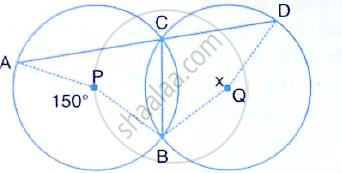Share

Books Shortlist

# In the Figure, Given Below, P and Q Are the Centres of Two Circles Intersecting at B and C Acd is a Straight Line. Calculate the Numerical Value of X. - ICSE Class 10 - Mathematics

ConceptArc and Chord Properties - the Angle that an Arc of a Circle Subtends at the Center is Double that Which It Subtends at Any Point on the Remaining Part of the Circle

#### Question

In the figure, given below, P and Q are the centres of two circles intersecting at B and C ACD is a straight line. Calculate the numerical value of x .#### Solution

∠ACB =  1/2 ∠APB =1/2 xx 150 = 75°
(Angle at the centre is double the angle at the circumference subtended by the same chord)
∠ACB + ∠BCD =180°
(Straight line)
⇒ ∠BCD =180° - 75° =105°
Also, ∠BCD =1/2 = reflex ∠BQD =1/2 (360° - x )

(Angle at the center is double the angle at the circumference subtended by the same chord) x
⇒ 105 = 180°
∴  x = 2 (180 ° -° ) = 2 ×75 = 150°

Is there an error in this question or solution?

#### Video TutorialsVIEW ALL 

Solution In the Figure, Given Below, P and Q Are the Centres of Two Circles Intersecting at B and C Acd is a Straight Line. Calculate the Numerical Value of X. Concept: Arc and Chord Properties - the Angle that an Arc of a Circle Subtends at the Center is Double that Which It Subtends at Any Point on the Remaining Part of the Circle.
S